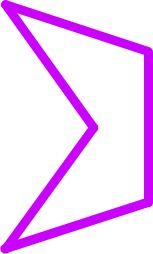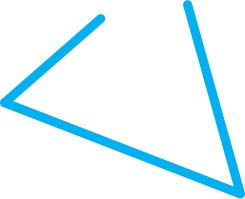Shapes and their Insides

Alignments to Content Standards: 3.MD.D

1. Draw a purple pentagon
2. Draw a blue shape with 3 line segments that is not a triangle.
3. Draw an orange shape with 4 line segments that is not a quadrilateral.
4. Draw a green triangle and color the inside blue.
5. Draw a red quadrilateral and color the inside orange.
6. The purple rectangle is drawn on a grid with squares that are 1 inch on each side. What is the perimeter of the rectangle? What is the area of the rectangle?7. What are you measuring when you find the perimeter of a rectangle? What are you measuring when you find the area of a rectangle?

IM Commentary

The purpose of this task is to help students differentiate between a polygon and the region inside of a polygon so that they understand what is being measured when the perimeter and area are being found.

A polygon is a figured composed of line segments that meet end-to-end, forming a simple closed curve. A polygon always divides the plane into exactly two regions - one "inside" the polygon and one "outside." In order to understand perimeter as an attribute of a plane figure, students need to be able to pick out the part of a figure that is being measured when finding perimeter. When finding the perimeter of a polygon, one finds the total length of all of the line segments that define the polygon. When finding the area, it is always the area of the region inside the polygon. It is common for students to get confused about which parts of the figure are part of the polygon itself (i.e. the line segments) and which are just defined by the polygon (i.e. the region inside it). One reason this is so confusing to students is that we ask students to "Find the perimeter and area of a rectangle," when in fact we mean, "Find the perimeter of a rectangle and the area of the region it encloses."

By drawing some non-examples, students can see why it doesn't make sense to try to find the area of a figure that is not closed--it isn't clear which region one would be measuring. In order for students to see this point, it will need to be made explicit in a class discussion after students have worked through the different parts of the task. If all of the examples students draw for parts (b) and (c) are closed, then it would be good to provide such an example when discussing part (g) as a class.

A variant of this task could ask students to draw a number of different polygons and to use the same color, such as blue, for all the line segments and then shade all the interior regions using a single color, such as red. This would help reinforce the difference between a region, which is measured by area, and the boundary of a region, which is measured by length.

Solution

There are many possibilities; here are some representative examples.

1.2.3.4.5.6. The perimeter of the rectangle is 3+4+3+4-14 inches. There are 3 rows with 4 square inches in each row inside the rectangle, so the area of the rectangle is $3\times4 = 12$ square inches.
7. When you find the perimeter of a rectangle, you are finding the total length of all the sides. In the picture, this means you are adding up the length of the 4 purple sides of the rectangle below.When you find the area of a rectangle, you are finding the total number of square units needed to cover the region inside the rectangle. That means finding the number of square units that cover the yellow region enclosed by the rectangle above.